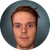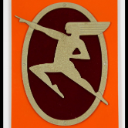# How to calculate geodesic area of polygons on map

1754
16
03-16-2020 06:11 AMby
Occasional Contributor III

Hi i want to calculate area of polygons drawn on map geometry, any arcgis sample will be helpful

1 Solution

Accepted SolutionsbyMVP Esteemed Contributor

Rajni,

A ring is just an array of coordinate and not an actual geometry class thus the geodesicArea method is failing.

Here is what you need to do instead.

``````      function calculateArea() {
var area = 0;
graphicsLayer.graphics.map(function (grap) {
area = geometryEngine.geodesicArea(grap.geometry, "hectares");
});
console.log(area);
} //End calculateArea‍‍‍‍‍‍‍‍‍‍‍‍‍‍``````
16 RepliesbyEsri Contributor

Take a look at the measure widget: Measurement in 2D | ArcGIS API for JavaScript 4.14byEsri Regular Contributor

There is also a lot of good information inside the GeometryEngine class:by
Occasional Contributor III

Hi Noah , I m trying to get geometry of all polygons from a map with below code, but doen't get rings area in console.

function calculateArea(){
graphicsLayer.graphics.map(function(grap){
var updatedGeometry = webMercatorUtils.webMercatorToGeographic(grap.geometry);
var rings_for_area = updatedGeometry.rings;
console.log(rings_for_area);//return all rings
});

var area = geometryEngine.geodesicArea(rings_for_area, "hectares");
console.log(area);//doesn't return area

}byMVP Honored Contributor

You have a scope problem. Since the variable rings_for_area is declared inside the map function, it's not available outside the function. Why aren't you calculating the area of the rings inside the map function and summing that up?by
Occasional Contributor III

Hi ken, this time i summed it up but due to this bolded code to calculate area, my whole map stops working. No error in console even. No area consoled even.

function calculateArea(){
var rings_for_area=[];
var k=0;
graphicsLayer.graphics.map(function(grap){
var updatedGeometry = webMercatorUtils.webMercatorToGeographic(grap.geometry);
var rings_area = updatedGeometry.rings;
rings_for_area= [];
rings_area.forEach(function(ring, i) {
rings_for_area=ring;
k++;
});
});
console.log(rings_for_area);
var area = geometryEngine.geodesicArea(rings_for_area, "hectares");
console.log(area);
}//End calculateAreaby
Occasional Contributor III

Do i need to convert them rings_for_area to  webMercatorUtils.geographicToWebMercator, Ok i have converted it and now it returns 0 area value, even when i have a polygon on map

function calculateArea(){
var rings_for_area=[];
var k=0;
graphicsLayer.graphics.map(function(grap){
var updatedGeometry = webMercatorUtils.webMercatorToGeographic(grap.geometry);
var rings_area = updatedGeometry.rings;
rings_for_area= [];
rings_area.forEach(function(ring, i) {
rings_for_area=ring;
k++;
});
});
console.log(rings_for_area);
var area = geometryEngine.geodesicArea( webMercatorUtils.geographicToWebMercator(rings_for_area), "hectares");
console.log(area);
}//End calculateAreabyMVP Honored Contributor

Since the geodesicArea method looks like it only takes a single polygon, try calculating that for each ring in your forEach loop

``````function calculateArea(){
var area = 0;
graphicsLayer.graphics.map(function(grap){
var updatedGeometry = webMercatorUtils.webMercatorToGeographic(grap.geometry);
var rings_area = updatedGeometry.rings;
rings_area.forEach(function(ring, i) {
area += geometryEngine.geodesicArea( webMercatorUtils.geographicToWebMercator(ring), "hectares");
});
});
console.log(area);
}//End calculateArea‍‍‍‍‍‍‍‍‍‍‍‍‍‍‍‍‍‍‍‍‍‍``````by
Occasional Contributor III

Hi ken , i used your code as it is, and it still returns 0. i called it here , do i need to call it somewhere else

//const vertices = <?php echo json_encode(\$map_ring_coordinate);?>;

const polygon = createGeometry(vertices);
validSymbol = createSymbol([255, 255, 255, 0.3], "solid", 2, [
255, 121,5]);
newDevelopmentGraphic = new Graphic({
geometry: webMercatorUtils.geographicToWebMercator(polygon),
symbol: validSymbol,
attributes: {
newDevelopment: "new store"
}
});
calculateArea();byMVP Esteemed Contributor

Rajni,

A ring is just an array of coordinate and not an actual geometry class thus the geodesicArea method is failing.

Here is what you need to do instead.

``````      function calculateArea() {
var area = 0;
graphicsLayer.graphics.map(function (grap) {
area = geometryEngine.geodesicArea(grap.geometry, "hectares");
});
console.log(area);
} //End calculateArea‍‍‍‍‍‍‍‍‍‍‍‍‍‍``````